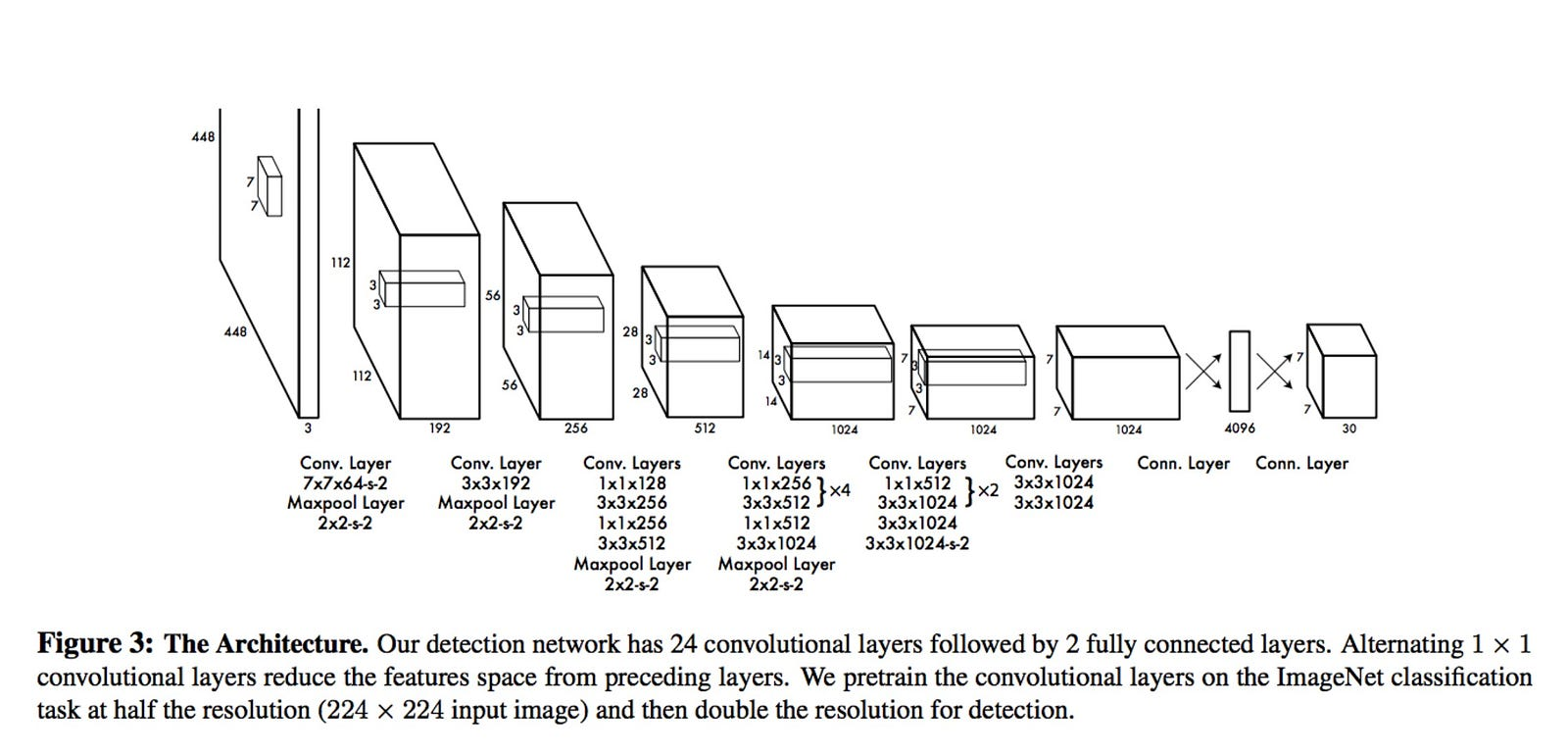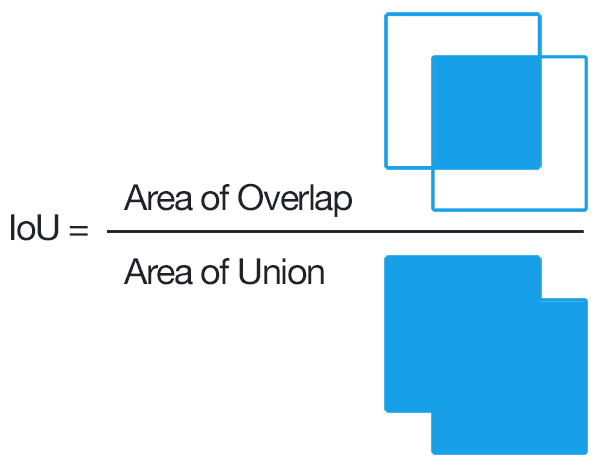# Can YOLO detect large objects?

I have a rather basic question about YOLO for bounding box detection.

My understanding is that it effectively associates each anchor box to an 8-dimension output.

During testing, does YOLO take each anchor box and classify on it alone? What happens if the object is big and spans over several anchor boxes (e.g., covering 70% of the image)? How can YOLO classify and detect objects spanning over many anchor boxes?

• If my memory is correct, I have seen objects larger than 70% of the image be detected. Have you tried to test on your own? Apr 14 '18 at 13:56
• @BrianO'Donnell Yes I have, but I'm just getting low f1 score for large objects. Apr 15 '18 at 19:23

The example given based on the yolov1 paper:The last layer has a tensor of the dimension 7x7x30. but the dimension of the last tensor is not in every case 7x7x30.

let be: S: the number of grid cells in X and Y direction C: the number classes to train B: the number of bounding boxes in every grid cell

The dimension of the output tensor is calculated with this formula: SxSx(5*B+C). The given example in their paper has the following values: S:7 B:2 C:20 (7X7X(5*2+20) = 7x7x30)

With this configuration you can detect at most 96 object (SSB -> 7*7*2) and 2 objects per grid cell. (e.g. it's not possible to detect many small object in a few grid cells)

now lets consider what are each feature map of the last tensor used for:

The data of every bounding box is stored in 5 feature maps: - 1 Feature map for the bounding box center in x direction - 1 Feature map for the bounding box center in y direction - 1 Feature map for the height of the bounding box - 1 Feature map for the width of the bounding box - 1 Feature map for the "confidence score" of the feature map

(confidence score = P(Object)*IoU(BoundingBox,Object))

• P(Object): the probability that in this cell is an object

• IoU: Intersection over Union. A ration between the overlapping area and the union area:additional there are a feature map for every class. In every cell the following probability is calculated: P(Cass|Object)

Long story short: If the bounding box is bigger than one grid cell, then some neurons share the same values (they share the bounding box center, the bounding box size and the confidence score, because they refer to the same bounding box).

edit no Bounding boxes on the image

if there are no bounding boxes on the image, then the confidence score would be zero, because there are no overlapping area. you can configure the threshold of the confidence score. look at this sample picture with threshold 0:additionally the feature maps of the classes would be zero because every neuron represents this probability P(Class|Object) because P(Class|Object) = P(Class And Object) / p(Object); (P(Class) = 0 -> P(Class and Object) = 0)

Is adding images without bounding boxes to the training set useful? I think it helps to lower the number of false positive matches. But that is just a guess. I have trained yolo to detect this plant https://en.wikipedia.org/wiki/Rumex_obtusifolius and I added a lot of picture without bouding boxes, becuase a low number of false positive matches was important. The result: the specificity was >99%.

Hope this helps

• Thanks for the explanation. Indeed, there's a feature map for every class. But is it useful to have images with no bounding boxes at all in the training set? Suppose there's only one class, does YOLO treat the images with no bounding boxes as negatives? Apr 15 '18 at 17:40